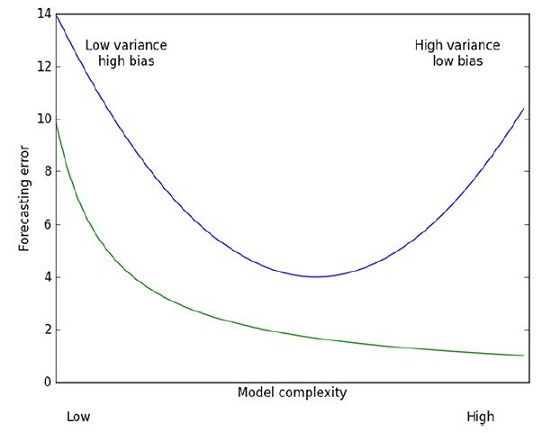## 2012年9月12日 星期三

### [ ML In Action ] Predicting numeric values : regression - Linear regression (3)

Preface :

Tikhonov regularization, named for Andrey Tikhonov, is the most commonly used method of regularization of ill-posed problems. In statistics, the method is known as ridge regression.

Ridge regression :Ridge regression was originally developed to deal with the problem of having more features than data points. But it can also be used to add bias into our estimations, giving us a better estimate. We can use the λ value to impose a maximum value on the sum of all our wsBy imposing this penalty, we can decrease unimportant parameters. This decreasing is known as shrinkage in statistics.

- regression.py :
1. def ridgeRegres(xMat,yMat,lam=0.2):
2.     xTx = xMat.T*xMat
3.     denom = xTx + eye(shape(xMat))*lam
4.     if linalg.det(denom) == 0.0:
5.         print "This matrix is singular, cannot do inverse"
6.         return
7.     ws = denom.I * (xMat.T*yMat)
8.     return ws
9.
10. def ridgeTest(xArr,yArr):
11.     xMat = mat(xArr); yMat=mat(yArr).T
12.     yMean = mean(yMat,0)
13.     yMat = yMat - yMean     #to eliminate X0 take mean off of Y
14.     #regularize X's
15.     xMeans = mean(xMat,0)   #calc mean then subtract it off
16.     xVar = var(xMat,0)      #calc variance of Xi then divide by it
17.     xMat = (xMat - xMeans)/xVar
18.     numTestPts = 30
19.     wMat = zeros((numTestPts,shape(xMat)))
20.     for i in range(numTestPts):
21.         ws = ridgeRegres(xMat,yMat,exp(i-10))
22.         wMat[i,:]=ws.T
23.     return wMat

wMat = zeros((numTestPts,shape(xMat)))

>>> ridgeWeights=regression.ridgeTest(abX, abY)
>>> ridgeWeights # 列印 λ=-10 的 ws
array([ 0.04304419, -0.02274163, 0.13214088, 0.02075182, 2.22403745,
-0.99895298, -0.11725424, 0.16622922])

>>> import matplotlib.pyplot as plt
>>> fig = plt.figure()
>>> ax.plot(ridgeWeights)
>>> plt.show()(x 軸為 λ 的 log 值; y 軸為 ws 的值. 不同顏色的線代表不同的 ws 欄位

Forward stagewise regression :1. def rssError(yArr,yHatArr): #yArr and yHatArr both need to be arrays
2.     return ((yArr-yHatArr)**2).sum()

1. def stageWise(xArr,yArr,eps=0.01,numIt=100):
2.     xMat = mat(xArr); yMat=mat(yArr).T
3.     yMean = mean(yMat,0)
4.     yMat = yMat - yMean     #can also regularize ys but will get smaller coef
5.     xMat = regularize(xMat)
6.     m,n=shape(xMat)
7.     returnMat = zeros((numIt,n)) #testing code remove
8.     ws = zeros((n,1)); wsTest = ws.copy(); wsMax = ws.copy()
9.     for i in range(numIt): # For each iteration
10.         print ws.T
11.         lowestError = inf;
12.         for j in range(n): # For each feature in ws
14.                 wsTest = ws.copy()
15.                 wsTest[j] += eps*sign
16.                 yTest = xMat*wsTest
20.                     wsMax = wsTest
21.         ws = wsMax.copy()
22.         returnMat[i,:]=ws.T
23.     return returnMat

>>> regression.stageWise(xArr, yArr, 0.01, 200) # 計算 200 次 iteration, 使用間距為 0.01
array([[ 0. , 0. , 0. , ..., 0. , 0. , 0. ],
[ 0. , 0. , 0. , ..., 0. , 0. , 0. ],
[ 0. , 0. , 0. , ..., 0. , 0. , 0. ],
...,
[ 0.05, 0. , 0.09, ..., -0.64, 0. , 0.36],
[ 0.04, 0. , 0.09, ..., -0.64, 0. , 0.36],
[ 0.05,
0. , 0.09, ..., -0.64, 0. , 0.36]])

>>> stageWiseMat = regression.stageWise(xArr,yArr,0.001,5000)(在 bottom 的曲線是 training error; 在 top 的曲線是 testing error

Supplement :
[ ML In Action ] Predicting numeric values : regression - Linear regression (1)
[ ML In Action ] Predicting numeric values : regression - Linear regression (2)
[ ML In Action ] Predicting numeric values : regression - Linear regression (3)

### [ Python 文章收集 ] Monitoring memory usage of a running Python program

Source From  Here Preface At  Survata , we do a lot of data processing using Python and its suite of data processing libraries like  pandas ...